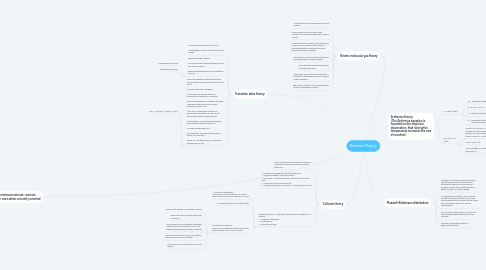# Kinetics TheoryNur Aiman Syazwani
Get Started. It's FreeKinetics Theory## 2. Arrhenius theory (The Arrhenius equation is founded on the empirical observation, that raising the temperature increases the rate of reaction)

### 2.1. k = Aexp(−E/RT)

2.1.1. Ea = activation energy

2.1.2. R = 8.314 J mol-1K-1

2.1.3. T = absolute temperature in Kelvin

2.1.4. A = frequency factor/Arrhenius constant/ pre-exponential factor

### 2.2. lnk= lnA+ (− E a/RT)

2.2.1. A plot of ln k versus 1/T produces a straight line, from which the activation energy, Ea, can be determined because the slope is equal to - Ea/ R.

2.2.2. Plot of ln k vs 1/T

2.2.3. Yields straight line slope = Ea/R intercept = A

## 5. Collision theory

### 5.3. Effective collision 1 that gives rise to product depends on 3 criteria i. Molecular orientation ii. Temperature iii. Activation energy

5.3.1. 1. Molecular Orientation Molecules must be oriented in a certain way during collisions for reaction to occur

5.3.2. 2. Temperature Greatly Affects Rates

5.3.3. 3. Activation Energy, Ea Molecules must possess certain amount of kinetic energy (KE) in order to react

5.3.3.1. Minimum KE needed for reaction to occur

5.3.3.2. Get energy from collision with other molecules

5.3.3.3. Upon collision, KE converted to potential energy (PE), used to stretch, bend, and break bonds leading to chemical reaction

5.3.3.4. If molecules move too slowly, too little KE, they just bounce off each other

5.3.3.5. Major reason all collisions do not lead to reaction

## 6. Transition state theory

### 6.2. Potential Energy Diagram To visualize what actually happens during successful collision Relationship between Ea and developing Total PE

6.2.1. Endothermic Reaction

6.2.2. Exothermic Reaction

### 6.3. H# is the enthalpy difference between the activated complex and the reactants A and B. It is called activation enthalpy S is for the entropy, the extent of randomness or disorder in a system. The entropy difference between activated complex and the reactants is called activation entropy S#. S# can be determined and thus is enabled the calculation of G# for the appropriate reaction temperatures A stabilization of the activated complex reduces the enthalpy difference  G# and increases the rate.  G# represents the determining driving power for a reaction. T he sign of G# determines if a reaction is spontaneous or not

6.3.1. lnkT = −ΔH†R1T + lnkBh + ΔS‡R